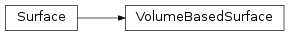# mvpa2.misc.surfing.volsurf.VolumeBasedSurface¶class `mvpa2.misc.surfing.volsurf.``VolumeBasedSurface`(vg)

A surface based on a volume, where every voxel is a node. It has the empty topology, meaning there are no edges between nodes (voxels)

Use case: provide volume-based searchlight behaviour. In that case finding neighbouring nodes is supposed to be faster using the circlearound_n2d method.

XXX make a separate module?

Attributes

`average_node_edge_length` Average length of edges associated with each face
`center_of_mass` Computes the center of mass
`edge2face` A mapping from edges to the face that contains that edge
`face_areas`
`face_edge_length` Length of edges associated with each face
`face_normals`
`faces`
`nanmean_face_normal`
`neighbors` Finds the neighbours for each node and their (Euclidean) distance.
`nfaces`
`node2faces` A mapping from node indices to the faces that contain those nodes.
`node_areas`
`node_normals`
`nvertices`
`vertices`

Methods

 `circlearound_n2d`(src, radius[, metric]) `connected_components`() `connected_components_slow`() `coordinates_to_box_indices`(box_size[, ...]) ‘Boxes’ coordinates into triples `dijkstra_distance`(src[, maxdistance]) Computes Dijkstra distance from one node to surrounding nodes `dijkstra_shortest_path`(src[, maxdistance]) Computes Dijkstra shortest path from one node to surrounding nodes. `dijkstra_shortest_path_visiting`(to_visit) Computes a list of paths that visit specific nodes `euclidean_distance`(src[, trg]) Computes Euclidean distance from one node to other nodes `map_to_high_resolution_surf`(highres[, ...]) Finds a mapping to a higher resolution (denser) surface. `map_to_high_resolution_surf_slow`(highres[, ...]) Finds a mapping to a higher resolution (denser) surface. `merge`(\*others) Merges the present surface with other surfaces `nearest_node_index`(src_coords[, ...]) Computes index of nearest node to src `nodes_on_border`([node_indices]) Determines which nodes are on the border of the surface `nodes_on_border_paths`() Find paths of nodes on the border `pairwise_near_nodes`([max_distance, src, trg]) Finds the distances between pairs of nodes `project_vertices`(n[, v]) Projects vertex coordinates onto a vector `rotate`(theta[, center, unit]) Rotates the surface `same_topology`(other) Returns whether another surface has the same topology `split_by_connected_components`() Splits a surface by its connected components `sub_surface`(src, radius) Makes a smaller surface consisting of nodes around a center node `vonoroi_map_to_high_resolution_surf`(highres_surf) Computes a Vonoroi mapping for the current (low-res) surface `write`(fn)
Parameters: vg: Volgeom.volgeom or str or NiftiImage volume to be used as a surface

Attributes

`average_node_edge_length` Average length of edges associated with each face
`center_of_mass` Computes the center of mass
`edge2face` A mapping from edges to the face that contains that edge
`face_areas`
`face_edge_length` Length of edges associated with each face
`face_normals`
`faces`
`nanmean_face_normal`
`neighbors` Finds the neighbours for each node and their (Euclidean) distance.
`nfaces`
`node2faces` A mapping from node indices to the faces that contain those nodes.
`node_areas`
`node_normals`
`nvertices`
`vertices`

Methods

 `circlearound_n2d`(src, radius[, metric]) `connected_components`() `connected_components_slow`() `coordinates_to_box_indices`(box_size[, ...]) ‘Boxes’ coordinates into triples `dijkstra_distance`(src[, maxdistance]) Computes Dijkstra distance from one node to surrounding nodes `dijkstra_shortest_path`(src[, maxdistance]) Computes Dijkstra shortest path from one node to surrounding nodes. `dijkstra_shortest_path_visiting`(to_visit) Computes a list of paths that visit specific nodes `euclidean_distance`(src[, trg]) Computes Euclidean distance from one node to other nodes `map_to_high_resolution_surf`(highres[, ...]) Finds a mapping to a higher resolution (denser) surface. `map_to_high_resolution_surf_slow`(highres[, ...]) Finds a mapping to a higher resolution (denser) surface. `merge`(\*others) Merges the present surface with other surfaces `nearest_node_index`(src_coords[, ...]) Computes index of nearest node to src `nodes_on_border`([node_indices]) Determines which nodes are on the border of the surface `nodes_on_border_paths`() Find paths of nodes on the border `pairwise_near_nodes`([max_distance, src, trg]) Finds the distances between pairs of nodes `project_vertices`(n[, v]) Projects vertex coordinates onto a vector `rotate`(theta[, center, unit]) Rotates the surface `same_topology`(other) Returns whether another surface has the same topology `split_by_connected_components`() Splits a surface by its connected components `sub_surface`(src, radius) Makes a smaller surface consisting of nodes around a center node `vonoroi_map_to_high_resolution_surf`(highres_surf) Computes a Vonoroi mapping for the current (low-res) surface `write`(fn)
`circlearound_n2d`(src, radius, metric='euclidean')